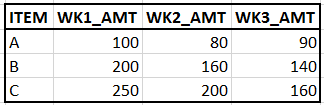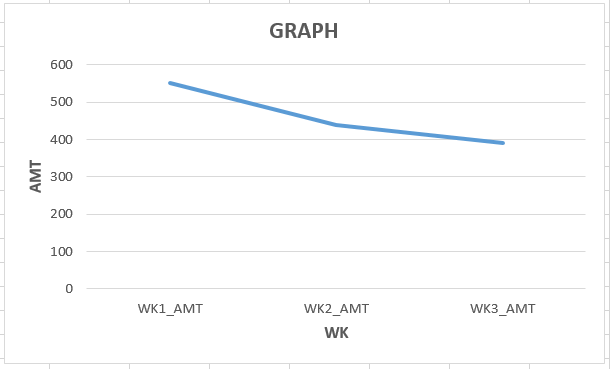# New to QlikView

Discussion board where members can get started with QlikView.

New Contributor III

## Using ValueList with Pick(Match(

Hi,

I am trying to create a graph from my existing data, but am having trouble in doing so. Here are the details.

I have a table that looks like this (not exactly, but the same idea for simplicity):I am trying to create a line graph, that puts sum(WK1_AMT), sum(WK2_AMT), & sum(WK3_AMT) on the same trend line. Just like this:As you can tell, its not so simple, since each amount represents its own column header/expression. My idea, and this is where my question lies, is if I can utilize PICK(MATCH(VALUELIST... where I create a value list of something like ValueList('WK1','WK2','WK3').

Then, in my expression, write something like:

PICK(MATCH(VALUELIST('WK1','WK2','WK3'),'WK1','WK2','WK3'),

sum(WK1_AMT),

sum(WK2_AMT),

sum(WK3_AMT)

))

Essentially, what I am trying to do, is force a manually created dimension value, to an expression. Trying to do the above, my graph keeps saying "No Data to Display", so I'm not sure if I am just writing it incorrectly, or if this just cannot be done (I did already add =Valuelist('WK1','WK2','WK3') in the calculated dimension.

Any help with trying to create the above graph, from the existing table would be greatly appreciated. I'm sure a suggestion of utilizing a crosstable would come up, but there is actually a lot more data in my table that would make that complicated. The above table is a simple idea of what I have.

Thank you all

1 Solution

Accepted Solutions
Contributor II

## Re: Using ValueList with Pick(Match(

Hi,

PFA the app with pick-match used in expression.

Thanks,

RT

6 Replies
Highlighted
Contributor III

## Re: Using ValueList with Pick(Match(

Hi,

Instead of Pick and match you can try if statement

=if(ValueList('WK1_AMT','WK2_AMT','WK3_AMT')='WK1_AMT', Sum(WK1_AMT),

if(ValueList('WK1_AMT','WK2_AMT','WK3_AMT')='WK2_AMT', Sum(WK2_AMT),

if(ValueList('WK1_AMT','WK2_AMT','WK3_AMT')='WK3_AMT', Sum(WK3_AMT))))

Please refer the attached qvw file

Thanks,

Pooja

Honored Contributor

## Re: Using ValueList with Pick(Match(

May be this:

=if(ValueList('WK1','WK2','WK3')='WK1', Sum(WK1_AMT),

if(ValueList('WK1','WK2','WK3')='WK2', Sum(WK2_AMT),

if(ValueList('WK1','WK2','WK3')='WK3', Sum(WK3_AMT),0)))

New Contributor III

## Re: Using ValueList with Pick(Match(

Hi,

Thanks for the response. However, I am trying to avoid using repeated IF statements, as because I have so much data in the table, it would really drag down the performance issues.

Any idea with a workaround on the IF statements?

Thank you

Contributor II

## Re: Using ValueList with Pick(Match(

Hi,

PFA the app with pick-match used in expression.

Thanks,

RTPartner

## Re: Using ValueList with Pick(Match(

PICK(MATCH(VALUELIST('WK1','WK2','WK3'),'WK1','WK2','WK3'),

sum(WK1_AMT),

sum(WK2_AMT),

sum(WK3_AMT)

))

I extra parentheses closing

New Contributor III

## Re: Using ValueList with Pick(Match(

This is it. Thank you!